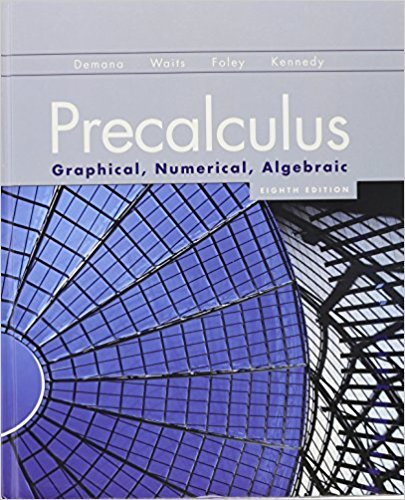×
×

# Solved: In Exercises 110, use NDER on a calculator to findISBN: 9780321656933 190

## Solution for problem 10.1.1.259 Chapter 10.4

Precalculus: Graphical, Numerical, Algebraic | 8th Edition

• Textbook Solutions
• 2901 Step-by-step solutions solved by professors and subject experts
• Get 24/7 help from StudySoup virtual teaching assistantsPrecalculus: Graphical, Numerical, Algebraic | 8th Edition

4 5 0 396 Reviews
15
2
Problem 10.1.1.259

In Exercises 110, use NDER on a calculator to find the numerical derivative of the function at the specified point.1x2 = x x = 1

Step-by-Step Solution:
Step 1 of 3

Experiment 32: Galvanic Cells Purpose: The purpose of this experiment is to measure the relative reduction potentials for a number of redox couples, develop and understanding of the movement of electrons, anions, and cations in a galvanic cell, study factors affecting cell potentials, and to estimate the concentration of ions in solution using the Nernst equation....

Step 2 of 3

Step 3 of 3

##### ISBN: 9780321656933

Precalculus: Graphical, Numerical, Algebraic was written by and is associated to the ISBN: 9780321656933. Since the solution to 10.1.1.259 from 10.4 chapter was answered, more than 210 students have viewed the full step-by-step answer. The answer to “In Exercises 110, use NDER on a calculator to find the numerical derivative of the function at the specified point.1x2 = x x = 1” is broken down into a number of easy to follow steps, and 25 words. This textbook survival guide was created for the textbook: Precalculus: Graphical, Numerical, Algebraic, edition: 8th Edition. The full step-by-step solution to problem: 10.1.1.259 from chapter: 10.4 was answered by , our top Calculus solution expert on 12/28/17, 04:31PM. This full solution covers the following key subjects: . This expansive textbook survival guide covers 88 chapters, and 6922 solutions.

Unlock Textbook Solution